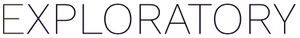# Extracting day, hour, min info from an hms column

#1

Currently, if you run “Convert to Numeric” on an hms column, it returns the same values regardless of the time unit. It is because the hms object always use ‘second’ for unit.

If you want to extract, day, hour or min info from an hms column, you can use the following formula at “Create Calculation” dialog.

• Day: `as.numeric(col) / (60 * 60 * 24)
• Hour: `as.numeric(col) / (60 * 60)
• Min: `as.numeric(col) / 60

1 Like
#2

Thanks for posting!

For the hours calculation, I get the correct answer when typing “as.numeric(hms_column)/(60 * 60)” or “as.numeric (hms_column)/ 60 / 60” as shown in the second image, but not “as.numeric(hms_column)/60 * 60” as the text above the second image suggests.

Thinking this syntax should apply to the Day column as well? “as.numeric(hms_column)/(60 * 60 * 24)” or “as.numeric (hms_column)/ 60 / 60/ 24”

#3

Thanks Stephanie.

Yes, you are right. The formula that I wrote looked weird because it conflicted with the markdown syntax. I updated my original post with your suggestion because it is more preciseThanks!
Kei